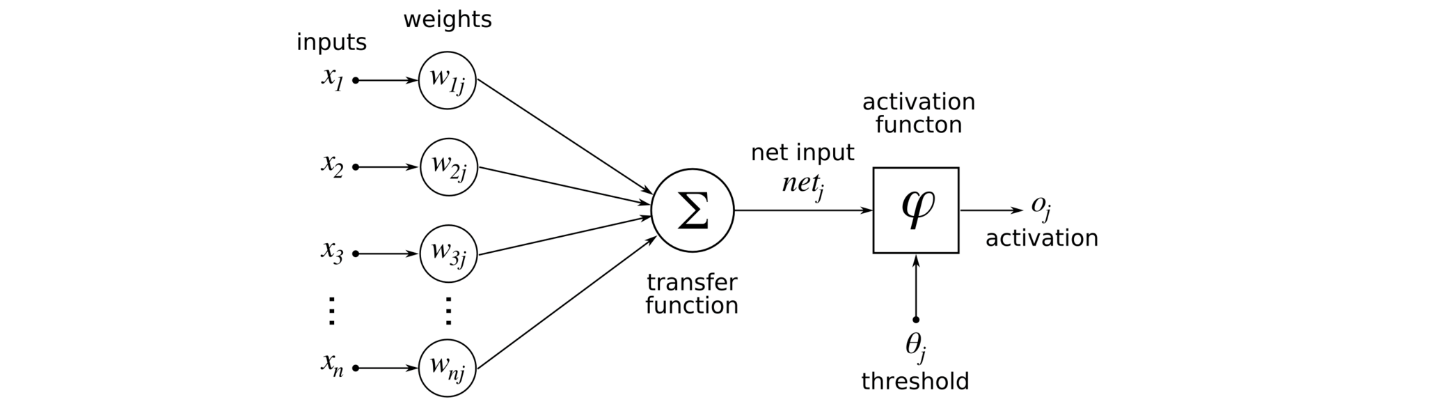/  Deep Learning Interview questions and answers   /  Explain Activation Function in Neural Network and its types.## Explain Activation Function in Neural Network and its types.

Ans: It is a Function used in Neural Network in order to calculate weighted sum and add bias with it. It also introduces non-linearity into output of a Neuron. Back Propagation is possible in Neural network by using Activation Function since the gradients are provided along with error to update the weights and biases. Due to no-linear transformation of input caused by Activation Function makes the Neural Network capable of Learning and Performing Complex tasks.

### Different types of Activation Functions:

• Binary Step
• Linear Activation
• Sigmoid/ Logistic
• Tanh / Hyperbolic Tangent
• ReLU (Rectified Linear Unit)
• Leaky ReLU
• Parametric ReLU
• Softmax
• Swish